• Slides: 19Quadratic Functions A Quadratic Function is an equation that has the form The graph of a Quadratic Equation is a u-shaped curve called a Parabola. The Vertex is the highest or lowest point of the parabola. The Vertex is also called the Turning Point. If a is positive, the parabola opens upward, and the vertex is the minimum point. If a is negative, the parabola opens downward, and the vertex is the maximum point. Maybe we should look at some diagrams.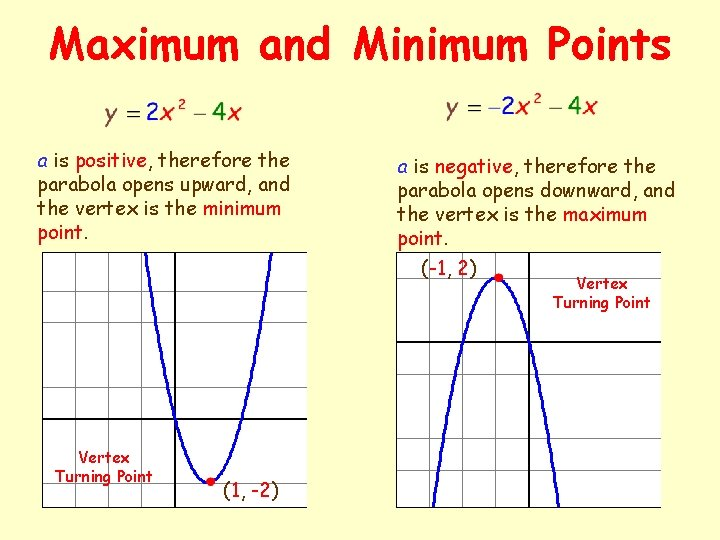Maximum and Minimum Points a is positive, therefore the parabola opens upward, and the vertex is the minimum point. a is negative, therefore the parabola opens downward, and the vertex is the maximum point. (-1, 2) Vertex Turning Point (1, -2) Vertex Turning Point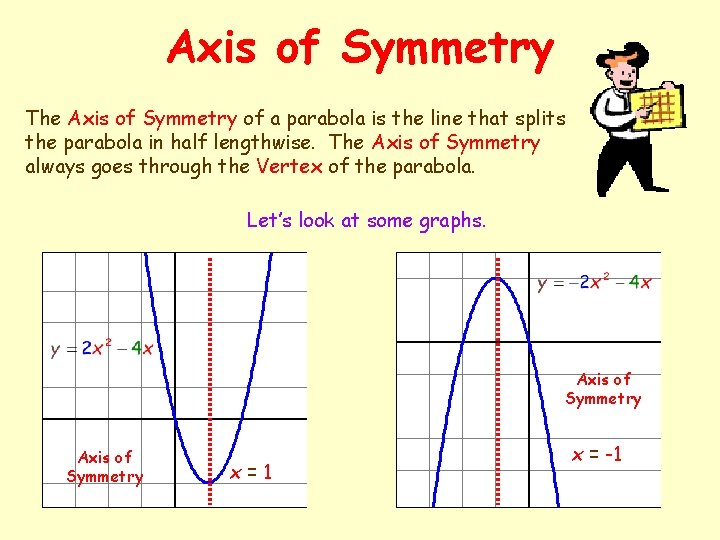Axis of Symmetry The Axis of Symmetry of a parabola is the line that splits the parabola in half lengthwise. The Axis of Symmetry always goes through the Vertex of the parabola. Let’s look at some graphs. Axis of Symmetry x=1 x = -1Finding the Axis of Symmetry You can find the Axis of Symmetry of any quadratic equation by using the formula Let’s take a look at those equations again. a = 2, b = -4, c = 0 vertex (1, -2) To find the coordinates of the vertex, plug the value of x into the original function. a = -2, b = -4, c = 0 vertex (-1, 2)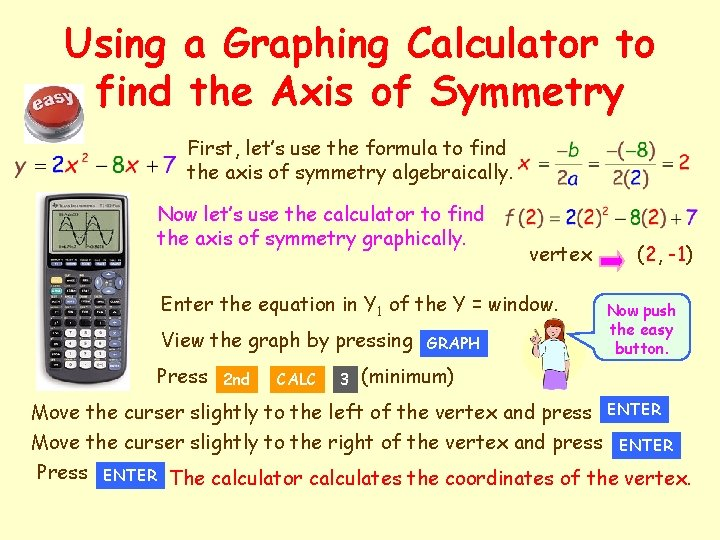Using a Graphing Calculator to find the Axis of Symmetry First, let’s use the formula to find the axis of symmetry algebraically. Now let’s use the calculator to find the axis of symmetry graphically. vertex Enter the equation in Y 1 of the Y = window. View the graph by pressing Press 2 nd CALC 3 GRAPH (2, -1) Now push the easy button. (minimum) Move the curser slightly to the left of the vertex and press ENTER Move the curser slightly to the right of the vertex and press ENTER Press ENTER The calculator calculates the coordinates of the vertex.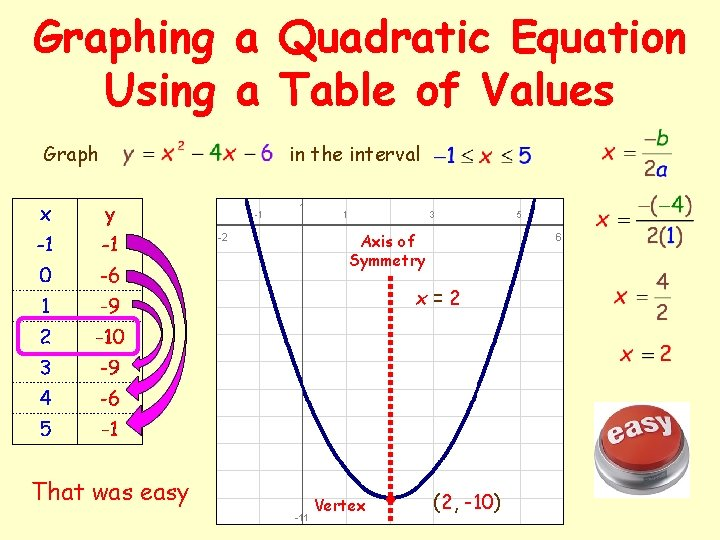Graphing a Quadratic Equation Using a Table of Values Graph in the interval Axis of Symmetry x=2 That was easy Vertex (2, -10)Graphing ExamplesQuadratic Functions Homework Page 156: 1 – 4, 6 Answer all questions on the graph paper. Show all your work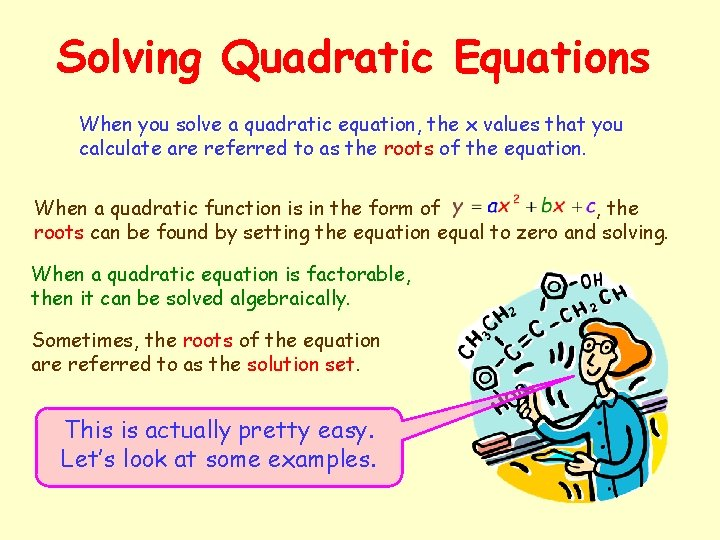Solving Quadratic Equations When you solve a quadratic equation, the x values that you calculate are referred to as the roots of the equation. When a quadratic function is in the form of , the roots can be found by setting the equation equal to zero and solving. When a quadratic equation is factorable, then it can be solved algebraically. Sometimes, the roots of the equation are referred to as the solution set. This is actually pretty easy. Let’s look at some examples.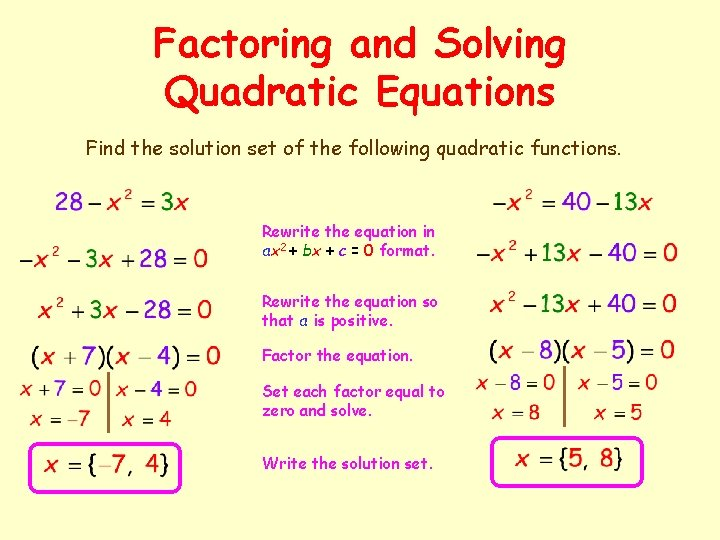Factoring and Solving Quadratic Equations Find the solution set of the following quadratic functions. Rewrite the equation in ax 2 + bx + c = 0 format. Rewrite the equation so that a is positive. Factor the equation. Set each factor equal to zero and solve. Write the solution set.More Factoring Examples For what values of x is the following fraction undefined? If the denominator was equal to zero, the fraction would be undefined. Solve the following equation for x Cross-multiply. Rewrite the equation in ax 2 + bx + c = 0 format. Factor the equation. The fraction would be undefined at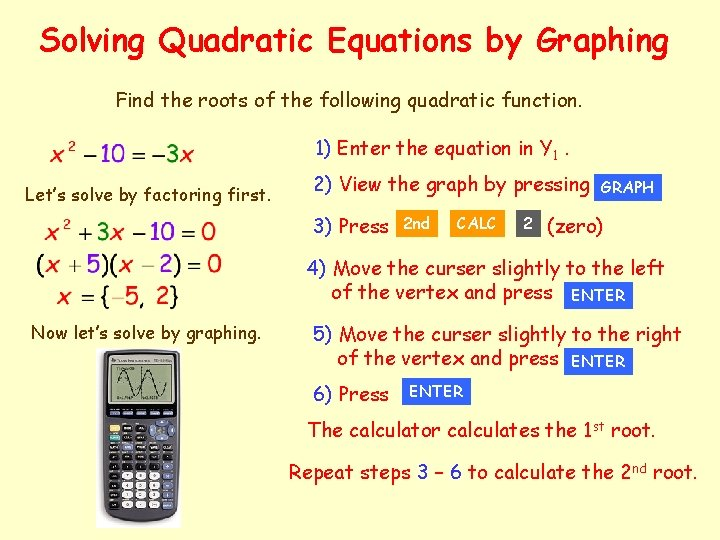Solving Quadratic Equations by Graphing Find the roots of the following quadratic function. 1) Enter the equation in Y 1. Let’s solve by factoring first. 2) View the graph by pressing 3) Press 2 nd CALC 2 GRAPH (zero) 4) Move the curser slightly to the left of the vertex and press ENTER Now let’s solve by graphing. 5) Move the curser slightly to the right of the vertex and press ENTER 6) Press ENTER The calculator calculates the 1 st root. Repeat steps 3 – 6 to calculate the 2 nd root.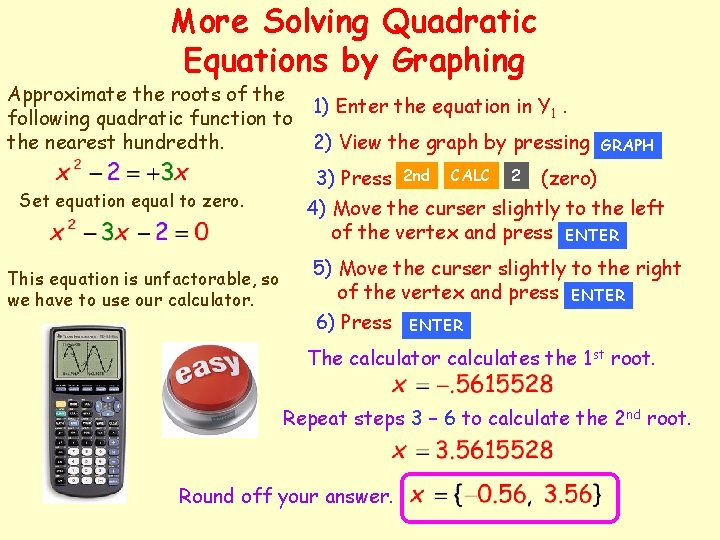More Solving Quadratic Equations by Graphing Approximate the roots of the following quadratic function to the nearest hundredth. Set equation equal to zero. This equation is unfactorable, so we have to use our calculator. 1) Enter the equation in Y 1. 2) View the graph by pressing GRAPH 3) Press 2 nd CALC 2 (zero) 4) Move the curser slightly to the left of the vertex and press ENTER 5) Move the curser slightly to the right of the vertex and press ENTER 6) Press ENTER The calculator calculates the 1 st root. Repeat steps 3 – 6 to calculate the 2 nd root. Round off your answer.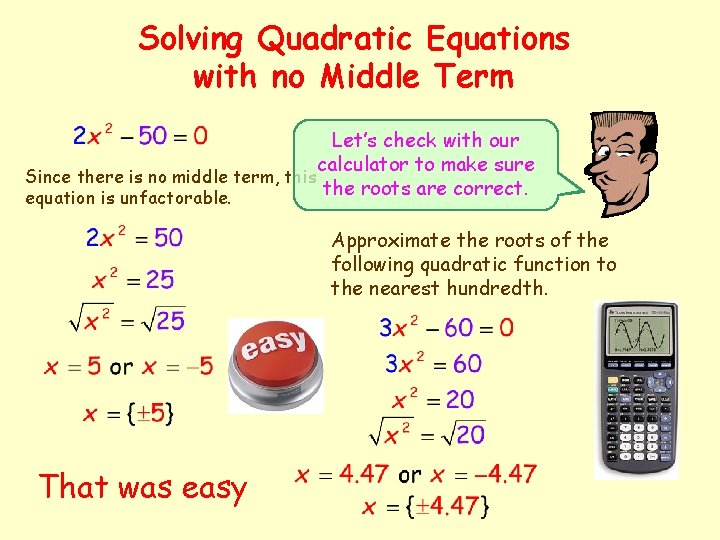Solving Quadratic Equations with no Middle Term Let’s check with our calculator to make sure Since there is no middle term, this the roots are correct. equation is unfactorable. Approximate the roots of the following quadratic function to the nearest hundredth. That was easy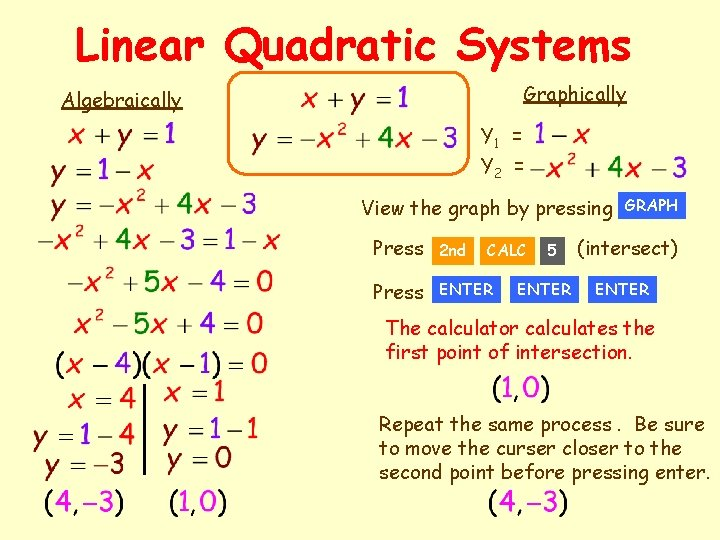Linear Quadratic Systems Graphically Algebraically Y 1 = Y 2 = View the graph by pressing Press 2 nd Press ENTER CALC 5 ENTER GRAPH (intersect) ENTER The calculator calculates the first point of intersection. Repeat the same process. Be sure to move the curser closer to the second point before pressing enter.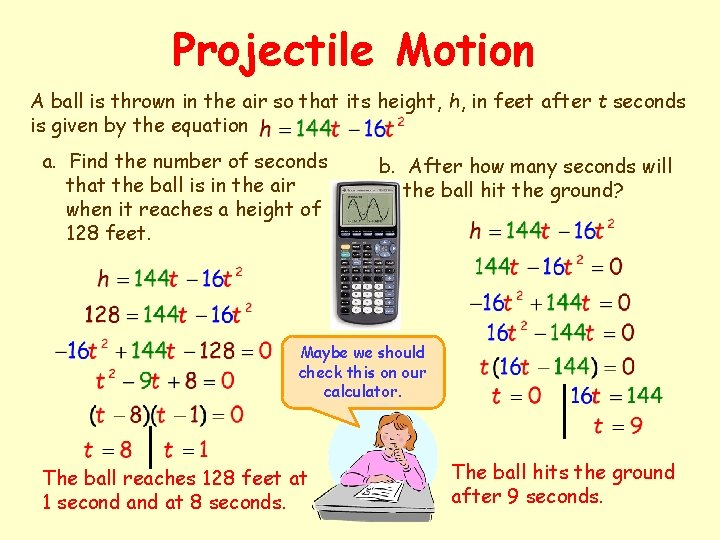Projectile Motion A ball is thrown in the air so that its height, h, in feet after t seconds is given by the equation a. Find the number of seconds that the ball is in the air when it reaches a height of 128 feet. b. After how many seconds will the ball hit the ground? Maybe we should check this on our calculator. The ball reaches 128 feet at 1 second at 8 seconds. The ball hits the ground after 9 seconds.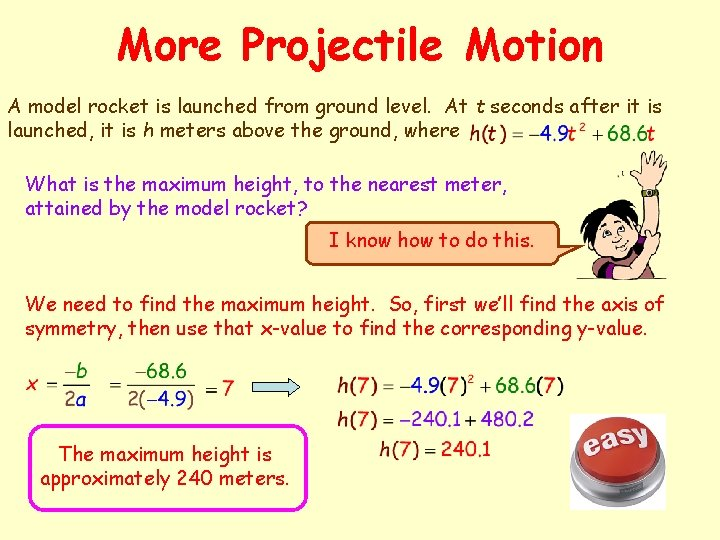More Projectile Motion A model rocket is launched from ground level. At t seconds after it is launched, it is h meters above the ground, where What is the maximum height, to the nearest meter, attained by the model rocket? I know how to do this. We need to find the maximum height. So, first we’ll find the axis of symmetry, then use that x-value to find the corresponding y-value. The maximum height is approximately 240 meters.Maximizing the Area of a Rectangle Stanley has 30 yards of fencing that he wishes to use to enclose a rectangular garden. If all the fencing is used, what is the maximum area of the garden that can be enclosed? x 15 - x Since all the fencing must be used, the perimeter of the garden will be 30 yards, and we can use the following equation. Let x represent the length and let w represent the width. Let A(x) represent the area of the rectangle. The maximum value occurs at The maximum area is 56. 25 yards 2.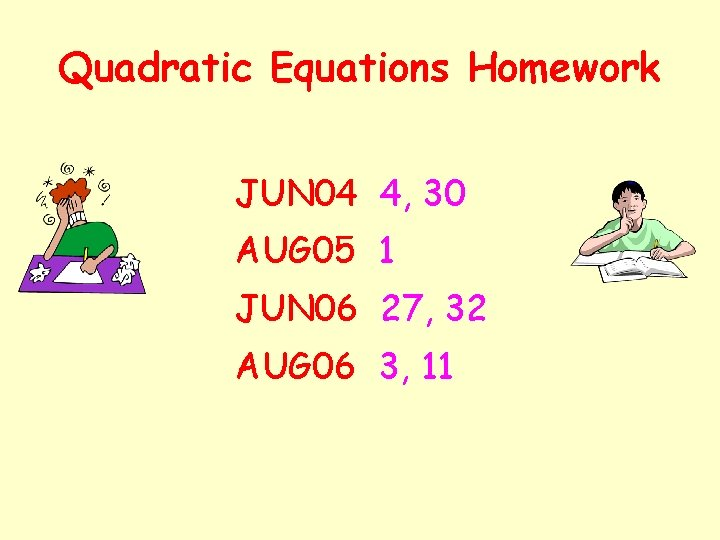Quadratic Equations Homework JUN 04 4, 30 AUG 05 1 JUN 06 27, 32 AUG 06 3, 11